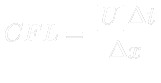## Iteration Output

• What are those iteration records?
• Lat us take a closer look at e.g. time 0.49 s:
```Time = 0.49
```

• Time means physical time in seconds
```Courant Number mean: 0.222158 max: 0.852134
```

• Courant Number mean/max is mean/maximal CFL number for convergence condition:(8.2)

```DILUPBiCG:  Solving for Ux, Initial residual = 2.09588e-07, Final residual = 2.09588e-07, No Iterations 0
```

• DILUPBiCG is linear system solver for U (Diagonal Incomplete LU Decomposition Preconditioned Bi-Conjugate Gradient)
• Linear system solver set-up is in file fvSolution
• Solver decomposes velocity vector into components and solves them separately
• Initial residual is the measure of stability of solution with respect to previous iteration.
• Final residual is the residual when linear system solver has finished.
• No Iterations is number of iterations of linear system solver
```DICPCG:  Solving for p, Initial residual = 1.0746e-06, Final residual = 3.53797e-07, No Iterations 1
```

• DICPCG is linear system solver used for pressure (Diagonal Incomplete Cholesky Preconditioned Conjugate Gradient)
• In PISO algorithm the pressure computation is repeated according to number of correctors nCorrectors
```time step continuity errors : sum local = 9.1113e-09, global = -6.21295e-19, cumulative = 1.23378e-18
```

• time step continuity errors represent certain measures of continuity equation violation
```ExecutionTime = 0.27 s  ClockTime = 0 s
```

• ExecutionTime is the time elapsed since the start of the computation
• ClockTime is the time spent on processor(s)